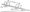# CSVTU Question Papers

## BE Engineering Mechanics June 2007

Q1 (a) A disabled automobile is pulled by two ropes as shown in fig 1.4 If the resultant of the two forces exerted by the ropes is 4 ken, which is parallel to the axis of the automobile ,find-

(i) The tension in each of the ropes knowing that a = 25°.

(ii) The value of a such the tension  in rope AC is minimum.(b) A Wheel of 60cm in diameter resting on the ground touches  to a rectangular block of 10cm height . Find the minimum pull required through the center of the wheel just to turn wheel over the corner of the block. Also , find the reaction of  the block to the wheel . Take weight to wheel = 1kN. Assuming  the all surfaces are smooth.

(c) The lever ABC of a component of a machine is hinged at  c and is subjected to a system of coplanar non-concurring  forces as shown in fig. Neglecting friction, determine magnitude of the forces F to keep the lever in equilibrium Also determine the magnitude and it reaction of the reactor at B.Q2 (a) Determine the forces in all members of the truss shown in fig using of joints.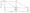(b) A plane truss is supported and loaded as shown in fig. find the axial force in members CD, EF and CF by method of sections.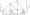(c) Draw the shear force and bending moment diagram for the simply supported load on right hand half of the span as shown in fig.Q3(a) A square threasded screw jack 8cm. mean diameter and cm pitch is used to lift a heavy load. The coefficient of the function at the treads . = 0.05

Find –

(i) The tangential force required at 40 cm radius to a i of 6 KN.

(ii) State whether the jack is self locking . if it is find the torque necessary to lower the load.

(iii)  But if not find the torque required to keep the load  from descending.

(b) A block B of weight 1000 N is being moved slowly the right by means of the wedge A under the action of the vertical force P. Find the value of P so as to just move the block if coefficient of friction at all surface of contacts is C neglect weight of the wedge.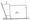(c) Block A shown in fig weight 2-400 N. the chord passes over a fixed drum and supports a we 1000N. The coefficient of friction between A and plane is 0.25 and that between the rope and the 0.12 solve for P if the motion is impending toward the right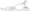Q4(a) find the moment of inertia of the section shown in fig. above horizontal and vertical axes through the centroid .

(b)Derive an expression for the moment of inertia of a triangul section about an axis passing through the center of gravity and parallel to the base.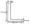(c) For the z-sextion shown in fig. determine the principal axes the  section about centoid.and the values of principal moment  inertia.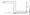Q5(a) A block of mass 5kg resting on a inclined plane is releases from rest. The block after traveling a distance elf 0.5m along inclined plane hits a spring of stiffness 15N/cm as shown in fig. 5.25. find the maximum compression of spring. Assume the coefficient of friction between the block and the inclined plane as 0.2.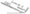(b) Two weights 80N and 29N are connected by a string as shown in fig and are lying on a rough horizontal on the 80N weight. Find the velocity of system after 2 second using impulse momentum principle. What is the tension in the string.(c) Two blocks A and B are held on an inclined plane 5m apart as shown in fig. The coefficients of friction between the blocks A and B and the inclined plane are 0.2 and 0.1 respectively. If the blocks begin to slide down the plane simultaneously, calculate the time and distance traveled by each block before collision.